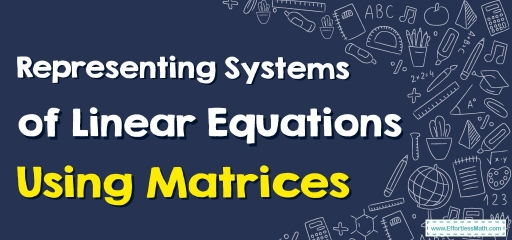# How to Represent Systems of Linear Equations Using Matrices?

A system of linear equations can be represented as a matrix. In this step-by-step guide, you learn how to represent systems of linear equations using matrices.A matrix equation consists of three matrices: the first matrix shows the values of the coefficients of the variables, the second lists the symbols of the variables themselves, and following an equal sign the third matrix represents the constant values to the right of the equal signs in the equations.

## A step-by-step guide to representing systems of linear equations using matrices

A system of linear equations can be represented as a matrix using a coefficient matrix, a variable matrix, and a constant matrix.

Consider the system:

$$\begin{cases}2x+5y=11 \\ 4x-2y=-26\end{cases}$$

The coefficient matrix can be formed by aligning the coefficients of the variables of each equation in a row. Make sure that each equation is written in the standard form with a constant expression on the right. Then the coefficient matrix for the above system:

$$\begin{bmatrix}2 & 5 \\4 & -2 \end{bmatrix}$$

The variables we have are $$x$$ and $$y$$. So we can write the variable matrix $$\begin{bmatrix}x \\y \end{bmatrix}$$.

To the right of the equation, we have the constant terms of equations, $$11$$ and $$-26$$. The two numbers correspond to the first and second equations, respectively, and therefore take the place of the first and second rows in the constant matrix. So, the matrix becomes $$\begin{bmatrix}11\\-26 \end{bmatrix}$$.

Now, the system can be displayed as:

$$\begin{bmatrix}2 & 5 \\4 & -2 \end{bmatrix}$$ $$+$$ $$\begin{bmatrix}x \\y \end{bmatrix}$$$$=$$ $$\begin{bmatrix}11\\-26 \end{bmatrix}$$

Using matrix multiplication we can see that the matrix representation is equivalent to the system of equations.

$$\begin{bmatrix}2 & 5 \\4 & -2 \end{bmatrix}$$ $$\begin{bmatrix}x \\y \end{bmatrix}$$ $$=\begin{bmatrix} 2(x) + 5(y) \\ 4(x) -2(y)\end{bmatrix}$$ $$=\begin{bmatrix} 2x + 5y \\ 4x -2y\end{bmatrix}$$

That is,  $$\begin{bmatrix} 2x + 5y \\ 4x -2y\end{bmatrix}$$$$=$$ $$\begin{bmatrix}11\\-26 \end{bmatrix}$$

Similarly, for a system of three equations in three variables:

$$\begin{cases}a_1x+b_1y+c_1z=d_1\\ a_2x+b_2y+c_2z=d_2\\ a_3x+b_3y+c_3z=d_3 \end{cases}$$

The matrix representation would be:

$$\begin{bmatrix}a_1 & b_1 & c_1\\ a_2 & b_2 & c_2\\ a_3 & b_3 & c_3 \end{bmatrix}$$ $$\begin{bmatrix}x \\ y \\ z \end{bmatrix}$$ $$=$$ $$\begin{bmatrix}d_1 \\ d_2 \\ d_3 \end{bmatrix}$$

We can generalize the result to $$n$$ variables.

### Representing Systems of Linear Equations Using Matrices – Example 1:

Represent the system of linear equations as a matrix. $$\begin{cases}3x-4y= -20 \\ -x+2y=10\end{cases}$$

solution:

$$\begin{bmatrix} 3 & -4 \\ -1 & 2\end{bmatrix}$$ $$\begin{bmatrix}x\\y \end{bmatrix}$$ $$=$$ $$\begin{bmatrix}-20\\10 \end{bmatrix}$$

### Representing Systems of Linear Equations Using Matrices – Example 2:

What linear system of equations does the matrix represent? $$\begin{bmatrix} 5& 2 \\ 0 & 1\end{bmatrix}$$ $$\begin{bmatrix}x\\y \end{bmatrix}$$ $$=$$ $$\begin{bmatrix}7\\9 \end{bmatrix}$$

solution:

$$\begin{bmatrix} 5& 2 \\ 0 & 1\end{bmatrix}$$ $$\begin{bmatrix}x\\y \end{bmatrix}$$ $$=$$ $$\begin{bmatrix} 5 (x) + 2(y) \\ 0(x) +1(y)\end{bmatrix}$$ $$=\begin{bmatrix} 5x+2y \\ 1y\end{bmatrix}$$

$$\begin{cases}5x+2y= 7 \\ y=9\end{cases}$$

## Exercises for Representing Systems of Linear Equations Using Matrices

### Represent the system of linear equations as a matrix.

1. $$\color{blue}{\begin{cases}x+y+z=6 \\ 2y+5z=-4 \\ 2x+5y-z=27 \end{cases}}$$
2. $$\color{blue}{\begin{cases}x+2y-4z=5 \\ 2x+y-6z=8 \\ 4x-y-12z=13 \end{cases}}$$
3. $$\color{blue}{\begin{cases}2v+x+2y+z=4 \\ v+5x-y-3z=1 \\ 4v-2x+6y-z=-16\\ 2v+6x-9z=10\end{cases}}$$
1. $$\color{blue}{\begin{bmatrix} 1 & 1 & 1 \\ 0 & 2 & 5 \\ 2 & 5 & -1 \end{bmatrix}\begin{bmatrix}x\\y \\z \end{bmatrix}=\begin{bmatrix}6\\-4\\ 27 \end{bmatrix}}$$
2. $$\color{blue}{\begin{bmatrix} 1 & 2 & -4 \\ 2 & 1 & -6 \\ 4 & -1 & -12 \end{bmatrix}\begin{bmatrix}x\\y \\z \end{bmatrix}=\begin{bmatrix}5\\8\\ 13 \end{bmatrix}}$$
3. $$\color{blue}{\begin{bmatrix} 2 & 1 & 2 & 1 \\ 1 & 5 & -1 & -3 \\ 4 & -2 & 6 & -1\\ 2 & 6 & 0 & -9 \end{bmatrix}\begin{bmatrix}v\\x\\y \\z \end{bmatrix}=\begin{bmatrix}4\\1\\ -16\\10 \end{bmatrix}}$$

### What people say about "How to Represent Systems of Linear Equations Using Matrices? - Effortless Math: We Help Students Learn to LOVE Mathematics"?

No one replied yet.

X
30% OFF

Limited time only!

Save Over 30%

SAVE $5 It was$16.99 now it is \$11.99